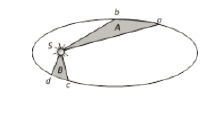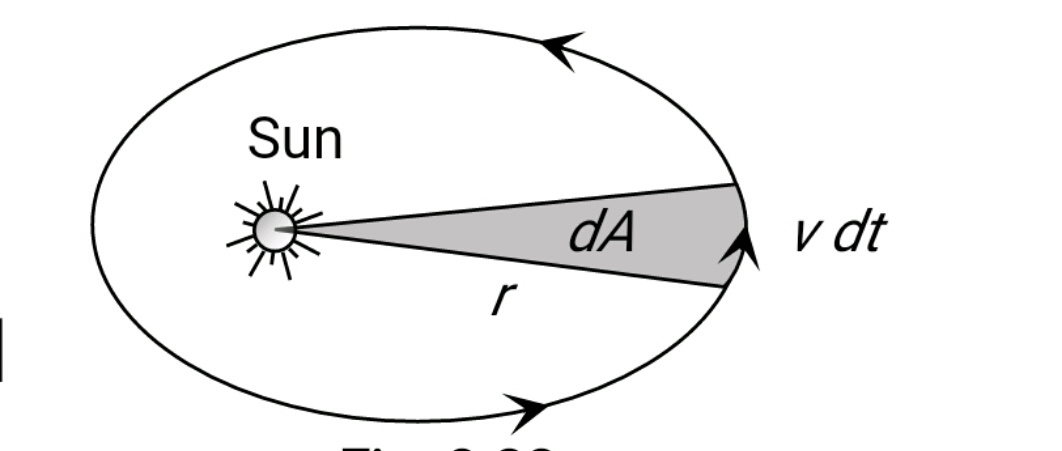#### The figure shows the motion of a planet around the sun in an elliptical orbit with sun at the focus. The shaded areas A and B are also shown in the figure which can be assumed to be equal. If t1 and t2 represent the time for the planet to move from a to b and d to c respectively, thenOption 1) t1 > t2 Option 2) t1 < t2 Option 3) t1 = t2 Option 4) none of theseAs we learnt in

Kepler's 2nd law -

Area of velocity =Areal velocitysmall area traced

- wherein

Simiar to Law of conservation of momentumAngular momentum

Known  as Law of Area

Areal velocity of planet in elliptical path is constant. This implies equal area will be covered in equal time.Option 1)

t1 > t2

This is incorrect option

Option 2)

t1 < t2

This is incorrect option

Option 3)

t1 = t2

This is correct option

Option 4)

none of these

This is incorrect option